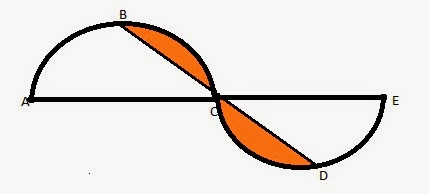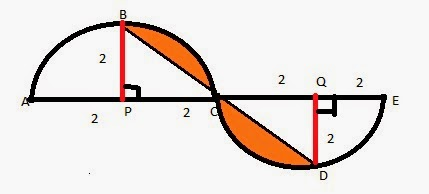# GMAT Problem Solving: Geometry

Q-51 Practice / Problem Solving / Geometry / Semicircles & Triangles / Q8
The core of what is tested is the ability to segregate the area of the semi circle into parts so that the area of the shaded region can be found.

## Question

• PS
• In the figure given below, ABC and CDE are two identical semi-circles of radius 2 units. B and D are the mid points of the arc ABC and CDE respectively. What is the area of the shaded region?A. 4π - 1
B. 3π - 1
C. 2π - 4
D. ½(3π - 1)
E. 2π - 2
• Correct Answer
Choice C. The area of the shaded region is 2π - 4

## Explanatory Answer

Click to access Explanatory Answer slide deck

## Detailed Solution

Segregate the semicircle into triangle and shaded region

P and Q are the centers of the two semicircles.

Draw BP perpendicular to AC.BP is radius to the semi-circle. So are AP and PC.

Therefore, BP = AP = PC = 2 units.

In semicircle ABC, area of the shaded portion is the difference between the area of half the semicircle PBC and the area of the triangle PBC.

Triangle PBC is a right triangle because PB is perpendicular to PC. PB and PC are radii to the circle and are equal. So, triangle PBC is an isosceles triangle.

Therefore, triangle PBC is a right isosceles triangle.

Compute areas of half the semicircle and the triangle## Area of half the semicircle - Area of region PBC

Area of the semicircle ABC = ½ area of the circle of radius 2.

So, area of half the semicircle, PBC = ¼ area of the circle of radius 2.

Area of half the semicircle, PBC = ¼ * π * 22

Area of half the semicircle, PBC = π sq units

## Area of right isosceles triangle PBC

Area of right triangle PBC = ½ PC * PB

Area of triangle PBC = ½ * 2 * 2 = 2 sq units

## Area of shaded region

Area of shaded region in one of the semi circles ABC = (π - 2) sq units

Therefore, area of the overall shaded region = 2(π - 2) sq units

Choice C is the correct answer.